Ch 4. Particle Momentum and Impulse Multimedia Engineering Dynamics Impulse & Momentum Consv. Linear Momentum Impact AngularMomentum MassFlow
 Chapter - Particle - 1. General Motion 2. Force & Accel. 3. Energy 4. Momentum - Rigid Body - 5. General Motion 6. Force & Accel. 7. Energy 8. Momentum 9. 3-D Motion 10. Vibrations Appendix Basic Math Units Basic Equations Sections Search eBooks Dynamics Fluids Math Mechanics Statics Thermodynamics Author(s): Kurt Gramoll ©Kurt GramollDYNAMICS - CASE STUDY SOLUTION Plastic Impact - Solution part a)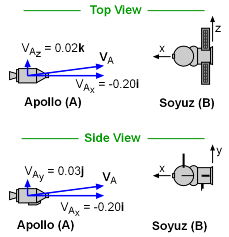Problem Diagram If the docking is successful, then the ships remain together, and the impact is perfectly plastic. Use the Conservation of Linear Momentum to solve for the velocity of the combined vehicles after the impact: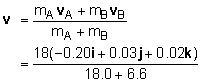= -0.146i + 0.022j + 0.015k m/s Although not needed for the plastic impact solution, it is interesting to note that for a perfectly plastic collision, the coefficient of restitution is zero.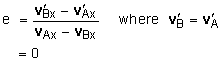Elastic Impact - Solution part b)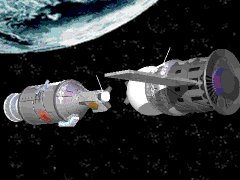Elastic Collision - Solid Animation If the docking is not successful, and the force of the impact is directed along the x-axis, then the collision is an oblique central impact. Since the force is only along the x-axis, then the Line of Impact will also be the x-axis. This also means that the y and z components of the velocity and momentum of each ship remain unchanged after the impact.      vAy = v'Ay = 0.03 m/s      vAz = v'Az = 0.02 m/s Apply the conservation of linear momentum in the x direction, gives,      mAvAx + mBvBx = mAv'Ax + mBv'Bx      18.0 (-0.2) = 18.0 v'Ax + 6.6 v'Bx This has two unknown, so a second equation or relationship is needed. Use the coefficient of restitution,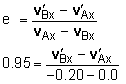-0.190 = v'Bx + v'Ax Solvign the two equations simultaneously gives,      v'Ax = -0.095       v'Bx = -0.285 Putting all values together gives,      vAx = -0.095i + 0.03j + 0.02k m/s      vBx = -0.285i m/s

Practice Homework and Test problems now available in the 'Eng Dynamics' mobile app
Includes over 400 problems with complete detailed solutions.
Available now at the Google Play Store and Apple App Store.